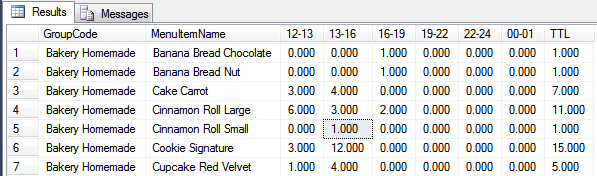# Burger Counter Per Hour

Hi! I run a small burger joint. In order to see if we’ve any choking points, i want to be develop a report to able to see how many burger we sell in a specific time period.

Currently i have the following report, but this just gives me a ticket count for a particular time period.I want to amend this report so that instead of giving a ticket count, it gives me a burger count.

I have a menu category “burgers”

first thing, you should not drill `TICKETS` table, you should apply this SQL to `ORDERS` table and filter it by MenuItems…

You can take a look at the `REPORT TAGS` topic, specifically `{REPORT ORDER DETAILS:<fields>:<expression>}` in:

G.

@gerlandog is correct, you want to query the ORDERS table. This should do it …

``````use [SambaPOS4]

SELECT
mi.[GroupCode]
,SUM(CASE WHEN (DATEPART(hour, [CreatedDateTime]) BETWEEN 12 AND 12) AND (DATEPART(minute, [CreatedDateTime]) BETWEEN 00 AND 59) THEN [Quantity] ELSE 0 END) as [12-13]
,SUM(CASE WHEN (DATEPART(hour, [CreatedDateTime]) BETWEEN 13 AND 15) AND (DATEPART(minute, [CreatedDateTime]) BETWEEN 00 AND 59) THEN [Quantity] ELSE 0 END) as [13-16]
,SUM(CASE WHEN (DATEPART(hour, [CreatedDateTime]) BETWEEN 16 AND 18) AND (DATEPART(minute, [CreatedDateTime]) BETWEEN 00 AND 59) THEN [Quantity] ELSE 0 END) as [16-19]
,SUM(CASE WHEN (DATEPART(hour, [CreatedDateTime]) BETWEEN 19 AND 21) AND (DATEPART(minute, [CreatedDateTime]) BETWEEN 00 AND 59) THEN [Quantity] ELSE 0 END) as [19-22]
,SUM(CASE WHEN (DATEPART(hour, [CreatedDateTime]) BETWEEN 22 AND 23) AND (DATEPART(minute, [CreatedDateTime]) BETWEEN 00 AND 59) THEN [Quantity] ELSE 0 END) as [22-24]
,SUM(CASE WHEN (DATEPART(hour, [CreatedDateTime]) BETWEEN 00 AND 00) AND (DATEPART(minute, [CreatedDateTime]) BETWEEN 00 AND 59) THEN [Quantity] ELSE 0 END) as [00-01]
,SUM(CASE WHEN (DATEPART(hour, [CreatedDateTime]) BETWEEN 12 AND 24) OR ((DATEPART(hour, [CreatedDateTime]) BETWEEN 00 AND 00) AND (DATEPART(minute, [CreatedDateTime]) BETWEEN 00 AND 59)) THEN [Quantity] ELSE 0 END) as [TTL]

FROM [Orders] o

WHERE 1=1
and [CreatedDateTime]>='{Start}'
and [CreatedDateTime]< '{End}'

``````P.S. the `BETWEEN` operator is inclusive, so when you do:

``````BETWEEN 12 AND 13
``````

and then

``````BETWEEN 13 AND 16
``````

anything that has a `DATEPART=13` will be counted for both `CASES`… I changed it in the above query so that it doesn’t double-count, like this:

``````,SUM(CASE WHEN (DATEPART(hour, [CreatedDateTime]) BETWEEN 12 AND 12) AND (DATEPART(minute, [CreatedDateTime]) BETWEEN 00 AND 59) THEN [Quantity] ELSE 0 END) as [12-13]

,SUM(CASE WHEN (DATEPART(hour, [CreatedDateTime]) BETWEEN 13 AND 15) AND (DATEPART(minute, [CreatedDateTime]) BETWEEN 00 AND 59) THEN [Quantity] ELSE 0 END) as [13-16]

etc...
``````

You should do the same for your Ticket query, as such:

``````SELECT
CONVERT(nvarchar(20),[Date],103)
,MIN(datename(dw,[Date])) as [WeekName]
,COUNT(CASE WHEN (DATEPART(hour, [Date]) BETWEEN 12 AND 12) AND (DATEPART(minute, [Date]) BETWEEN 00 AND 59) THEN 1 ELSE 0 END) as [12-13]
,COUNT(CASE WHEN (DATEPART(hour, [Date]) BETWEEN 13 AND 15) AND (DATEPART(minute, [Date]) BETWEEN 00 AND 59) THEN 1 ELSE 0 END) as [13-16]
,COUNT(CASE WHEN (DATEPART(hour, [Date]) BETWEEN 16 AND 18) AND (DATEPART(minute, [Date]) BETWEEN 00 AND 59) THEN 1 ELSE 0 END) as [16-19]
,COUNT(CASE WHEN (DATEPART(hour, [Date]) BETWEEN 19 AND 21) AND (DATEPART(minute, [Date]) BETWEEN 00 AND 59) THEN 1 ELSE 0 END) as [19-22]
,COUNT(CASE WHEN (DATEPART(hour, [Date]) BETWEEN 22 AND 23) AND (DATEPART(minute, [Date]) BETWEEN 00 AND 59) THEN 1 ELSE 0 END) as [22-24]
,COUNT(CASE WHEN (DATEPART(hour, [Date]) BETWEEN 00 AND 00) AND (DATEPART(minute, [Date]) BETWEEN 00 AND 59) THEN 1 ELSE 0 END) as [00-01]

FROM [Tickets]

WHERE 1=1
and [DepartmentId] in (select [Id] from [Departments] where [Name] = '@1')
and [Date] > '{Start}' and [Date] < '{End}'

GROUP BY Convert(nvarchar(20),[Date],103)``````
2 Likes

Thanks - will make the necessary changes to the ticket count file. For the burger count file, i’m getting the following error:

There was a typo - it was `by by` - I corrected it in the original post to a single `BY`